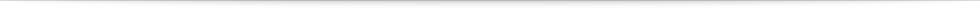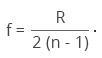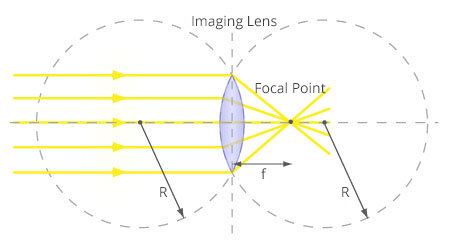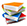Teach
With
Bigshot
 Prev Introduction      Pinhole      Refraction      Lens Basics      Thin Lens Lens Properties      Focusing      Image Appearance      Illustrations Next### Properties of a Lens

Aperture: The total light-receiving area of the lens is called its aperture . The larger the aperture, the greater the amount of light received by the lens from each point in the scene, and hence the brighter the image. The size of the aperture (opening) can be controlled using a manually adjustable diaphragm, or iris, which is placed right next to the lens. This enables us to increase the aperture size when photographing dark objects, and make the aperture smaller when photographing very bright objects. The iris of a camera lens therefore serves the same purpose as the iris of the human eye. Use the buttons in Figure 10 to see how the iris of a lens works.

 Figure 10: Changing aperture of a lens using an iris

Focal Length: Figure 11 shows a simple convex lens. Each of the lens's two surfaces is part of a sphere of radius R. The dotted line that connects the centers of these spheres is called the optical axis. The lens converges incoming light rays that are parallel to the optical axis toward a single point on the optical axis called the focal point. The distance between the focal point and the center of the lens is called the focal length (f)  of the lens. It is a measure of the focusing power of the lens and is related to the radius of curvature (R) and refractive index (n) of the lens as follows :Thicker lenses with a shorter radius of curvature R have a shorter focal length, while thinner lenses with a longer radius of curvature R have a longer focal length. The focal length is the most important property of a lens and it determines where exactly the image is formed.Figure 11: Focal point of a convex lens
 Prev Next###References

  "Aperture" Wikipedia, The Free Encyclopedia. Sept 12, 2012. [Online]. Available: http://en.wikipedia.org/wiki/Aperture.  "Focal Length," Wikipedia, The Free Encyclopedia. Aug 3, 2012. [Online]. Available: http://en.wikipedia.org/wiki/Focal_length.  E. Hecht, Optics. Addison Wesley, 2001.